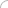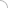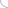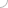# Scientific Visualization interview questions and answers

## Scientific Visualization interview questions and answers

### 1. What do you understand by visualization? 2. What is scientific visualization? How is it different from visualization? 3. What is the main purpose of scientific visualization? 4. Give a brief case study on applications of visualization in field of science. 5. What is the significance of IT industry over scientific visualization? 6. Name few of the popular scientific visualization techniques. 7. What are the tools available in the market these days that are suitable for scientific visualization? 8. Differentiate visualization with statistical graphics & infographics. 9. Explain the downsides of visualization compared to other techniques. 10. Is it possible to create interactive visualization? Which tool is best in such scenarios? 11. Draw the flowchart of scientific visualization stages. 12. What are the primitive forms of data representation? 13. What do you understand by computer simulation? How can it help a scientist in visualization? 14. What is information visualization? Is there any difference between data visualization & information visualization? 15. What is volume visualization? How data can be visualized using it? 16. What is ray casting? How it helps in surface rendering? 17. Give a scenario in which radiosity or global illumination plays an important role. 18. What is Ray tracing? How is it different from ray casting? 19. If there is a scenario in which you have visualize a multi dimensional dataset, which visualization technique you would prefer to use? 20. In case of huge datasets to be visualized which technique is best suited? 21. Name few business cases in field of geography that can make use of scientific visualization to assist them in their work. 22. How is scientific visualization different from mathematical visualization? 23. What is the main problem faced by professionals in field of visualization? 24. How modeling of color is done? 25. Explain RGB & HSV color models. 26. How data is mapped to the colors? 27. Explain the taxonomy of visualization techniques. 28. How will you plot 1D data? Give few examples of techniques one can use to map scientific data. 29. What is a Histogram? 30. Mention few of the techniques that can be used to map 2D data. 31. What is a contour? What are its applications? 32. For what purposes a Height field plot can be used? 33. What are Isosurfaces? What is their significance? 34. How can one visualize 3D data over 2D plane? 35. What are the visualization vectors? Explain Arrow Plot. 36. What are Streamribbon, Streamsurface, and Streamtube? 37. What is a surface view? How is it different from contours? 38. What are the applications of 2D histogram? 39. What do you mean by interpolation? How it helps in visualization? 40. What do you mean by surface interrogation & modeling? 41. What is the best technique that can be used to map whole visible universe? 42. What are the design implications that must be kept in mind while planning for visualization? 43. What is scientific visualization glossary? 44. What role does image analysis play in medical visualization? 45. What do you understand by volume visualization? 46. What do you mean by virtual endoscopy? How is it helpful? 47. How visualization helps in education? (adsbygoogle = window.adsbygoogle || []).push({}); Write your comment - Share Knowledge and Experience (adsbygoogle = window.adsbygoogle || []).push({}); (adsbygoogle = window.adsbygoogle || []).push({});Interview questionsLatest MCQs » General awareness - Banking » ASP.NET » PL/SQL » Mechanical Engineering » IAS Prelims GS » Java » Programming Language » Electrical Engineering » English » C++ » Software Engineering » Electronic Engineering » Quantitative Aptitude » Oracle » English » Finance Home | About us | Sitemap | Contact us | We are hiring © Copyright 2016. All Rights Reserved. Terms of use  |  Follow us on Facebook!